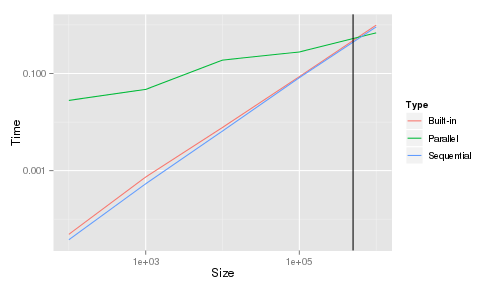# M2R MOSIG/PDES: First steps in evaluating the effect of using threads on performance

This document is a simple template to illustrate the kind of report we expect from you (for now…). I follow the classical IMRAD structure. Obviously, there is a lot to improve in both the study and the document but that's the whole point… :)

# Introduction

The goal of this study is to evaluate when using threads becomes interesting compared to a simple sequential version. To this end, we use the well-known quicksort algorithm whose parallelization is rather natural.

# Method

We used our own machine, a Dell Latitude 6430u with 16Gb of RAM. Everything was provided in the archive so we simply launched the measurement by calling `make`.

# Results

Let's first load the obtained measurement.

``````library(ggplot2)
``````

Let's compute the average execution time for each size and type of measurement.

``````library(plyr)
df_avg <- ddply(df, c("Size", "Type"), summarise, Time = mean(Time))
``````

And finally, let's plot it.

``````ggplot(data = df_avg, aes(x = Size, y = Time, color = Type)) + geom_line() +
scale_x_log10() + scale_y_log10() + geom_vline(xintercept = 5e+05)
``````As can be seen thanks to the previous analysis, activating parallelism becomes interesting as soon as tables comprise 5E05 elements, which answers our initial question.

# Discussion

Multi-core machines are definitely the best answer to the evergrowing needs of performance. Our study illustrates however that performance gains can be obtained even for data sets. Yet, we however that even better performances could be obtained and we intend to investigate this question in future work.